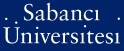# Curves of every genus with a prescribed number of rational points

Anbar, Nurdagül and Stichtenoth, Henning (2013) Curves of every genus with a prescribed number of rational points. Bulletin of the Brazilian Mathematical Society, 44 (2). pp. 173-193. ISSN 1678-7544 (Print) 1678-7714 (Online)

Full text not available from this repository.

Official URL: http://dx.doi.org/10.1007/s00574-013-0008-8

## Abstract

A fundamental problem in the theory of curves over finite fields is to determine the sets M (q) (g):= {N a a"center dot / there is a curve over of genus g with exactly N rational points}. A complete description of M (q) (g) is out of reach. So far, mostly bounds for the numbers N (q) (g):= maxM (q) (g) have been studied. In particular, Elkies et al. proved that there is a constant gamma (q) > 0 such that for any g a parts per thousand yen 0 there is some N a M (q) (g) with N a parts per thousand yen gamma (q) g. This implies that lim inf (g -> a) N (q) (g)/g > 0, and solves a long-standing problem by Serre. We extend the result of Elkies et al. substantially and show that there are constants alpha (q) , beta (q) > 0 such that for all g a parts per thousand yen 0, the whole interval [0, alpha (q) g - beta (q) ] a (c) a"center dot is contained in M (q) (g).

Item Type: Article Curve; function field; finite field; genus; rational point; Hasse-Weil bound Q Science > QA Mathematics 21987 Henning Stichtenoth 07 Nov 2013 16:24 01 Aug 2019 10:56

Repository Staff Only: item control page